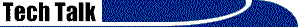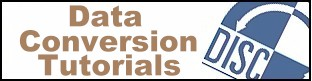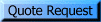# Character, BCD, and Binary fields

This brief technical article explains the differences between character, BCD, and binary numeric fields in a data file.  The intended audience are those people with limited computer knowledge, who are unfamiliar with these types of fields but wish to have a basic understanding of how data is stored in a file.  This is a general and simplistic overview, and it makes no attempt to cover all types of binary fields.  This article is primarily describing the format of the data that is stored in a file on your hard disk.  Internal representation of these values while in memory is not necessarily the same.

## Character Fields

Character or alpha-numeric fields are probably what you are most familiar with, so we will start with a description of this field type.  We are using the term "character" to denote a character of the alphabet, such as "A", "B", "C". "a", "b", "c", or "1", "2" ,"3" encoded using the ASCII (or EBCDIC) code set.  When we are discussing numeric fields in this context, references to "characters" will generally mean numbers, spaces, "+", "-", and "." (the decimal point).

A character field containing numbers is simply a text field.  It stores the number in the disk file as a text character, using the ASCII (or EBCDIC) code for that character, just as-if you had typed it using a text editor.  The number is stored in base-ten notation using characters, just like you typed it.  For example, the value 123 is stored as three characters: "1", "2", "3".  Since each character is coded into one 8-bit byte, storing the value this way requires one byte per digit, plus a byte for the sign and a byte for the decimal point.

Because this type of field is stored as text, it can be viewed with a text editor or simply typed to the screen, and it will display as the characters "123", just like the letters of the alphabet would display as "ABC...".

A field containing numbers stored this way can be defined as either an "alpha" field or a "numeric" field.  The definition doesn't change how the data is stored on disk -- in all cases the data is stored as ASCII (or EBCDIC) characters -- but the "numeric" definition restricts the legal values of the field to numbers, sign, and decimal point.  An "Alpha" field can contain any letter of the alphabet.  But, of course, if there were a letter in that field, it would not be a valid numeric value.

That's about all there is to character fields -- they are just plain text.

## BCD Fields

In the discussion of Character Fields, above, we said that numbers, such as 123, were stored in the file in decimal (base ten) notation.  In the case of character fields, each digit of the base ten number is stored in character representation using ASCII (or EBCDIC) coding, which requires 8 bits (1 byte) per digit.  However, there are only ten digits in the base ten number system, 0-9, and ten values can be represented by just 4 bits -- half of the 8 bits required for character storage. (4 bits can represent up to 16 different values)  If we only use 4 bits to represent a digit, we can now store two digits in 8 bits, or one byte.

This is the concept behind BCD, or Binary Coded Decimal.  The name "Binary Coded Decimal" actually describes the storage method - Decimal representation which is coded in binary.

Here's how it works:  The value you want to store, say 1234, is represented in decimal (base 10) notation (as opposed to binary representation).  Then, each of the decimal digits is independently coded using a 4 bit binary value.  The independent coding of each digit is what makes this different than straight binary.

So, each of the digits in the value 1234 would be individually coded as:

```Decimal  Binary
=======  ======
1       0001
2       0010
3       0011
4       0100
```

And the final result in binary is: 0001  0010  0011  0100.  Notice each of these decimal digits is represented by four bits.  Since each byte is 8 bits, we can get exactly two decimal digits in one byte.  Placing these four values into two bytes results in:  00010010   00110100.  We have now stored four digits in just two bytes, half the space required by a character field.

The difference between binary and BCD storage is that binary stores the entire value as a single binary number, whereas BCD encodes each digit independently.  The results are not the same.  For example, here's the value 1234 stored in binary and BCD format, using 2 bytes:

```Decimal: 1234
Binary:  00000100   11010010
BCD:     00010010   00110100
```

In the real world, most values need a sign and a decimal point.  The sign is commonly stored in the last 4 bit nybble of the value, in place of a digit, and the decimal point is usually implied, not real.  These are further discussed in our articles COBOL Comp-3 Packed fields and Implied Decimal.

 Need to convert Binary fields?That's our business!

## Binary Fields

There are many kinds of binary fields, but for this article we will only discuss unsigned integer fields.  In contrast to BCD, which encodes each digit separately (see above), a pure binary field stores the entire value as a single base 2 number.

Binary fields vary in size, depending on the largest value the field is required to contain.  Field sizes are usually some multiple of 8 bits, because of current CPU designs, but this is not mandatory.  For unsigned values, an 8 bit field can hold values from 0 - 255, a 16 bit field can hold values from 0 - 65,535, a 32 bit field can hold values from 0 - 4,294,967,295, etc.  An important concept to understand is that this field, say a 32 bit unsigned binary integer, is a single 32 bit value, regardless of the word size of the CPU.  If the CPU is, say, 16 bits, then it will have to make two memory accesses to load the 32 bit number, but it will still perform 32 bit computations on the number, not 16 bit computations.

Here are some values represented as 16 bit unsigned integers.  The most significant bit is on the left:

```16 bit binary value    Decimal equivalent
===================    ==================
00000000  00000000            0
00000000  00000001            1
00000000  00000010            2
00000000  00000011            3
00000000  00000100            4
00000000  00001000            8
00000000  00001001            9
00000000  11111111          255
00000001  00000000          256
00000001  00000001          257
00000001  00000010          258
00000010  00000000          512
00000100  00000000         1024
10000000  00000000        32768
11111111  11111111        65535
```

## Summary

1. Character fields store the value as text.
2. BCD separately encodes each digit into binary and stores the result.
3. Binary fields store the value as a single base 2 binary number.

Here is the binary representation of the value 1234 in each of these field types, as stored in 4 bytes.  The character representation is coded in ASCII. The most significant bit is on the left, and none of these fields has a sign.

```Mode         Byte 4    Byte 3     Byte 2     Byte 1
=========   ========   ========   ========   ========
Character   00110001   00110010   00110011   00110100
BCD         00000000   00000000   00010010   00110100
Binary      00000000   00000000   00000100   11010010
```

Notice that the character representation requires 4 bytes, the BCD needs 2 bytes, and the binary easily fits in 2 bytes .

Two bytes of storage can hold the following maximum unsigned values:

```Character field:   99
BCD field:       9999
Binary field:   65535
```

This ratio varies depending on the value, but this demonstrates the savings that binary and BCD offer.